# High School Math : How to find the length of the diameter

## Example Questions

### Example Question #131 : Plane Geometry

What is the diameter of a circle with a circumference of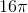?

Possible Answers: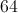Correct answer:Explanation:

To find the diameter we must understand the diameter in terms of circumference. The equation for the circumference of a circle is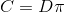, whereis the circumference andis the diameter. The circumference is equal to the diameter multiplied by.

We can rearrangeto solve for.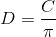All we have to do is plug in the circumference and divide by, and it will yield the diameter.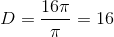Thes cancel and the diameter is.

### Example Question #1 : How To Find The Length Of The Diameter

If the area of a circle is four times larger than the circumference of that same circle, what is the diameter of the circle?

Possible Answers:

4

32

8

16

2

Correct answer:

16

Explanation:

Set the area of the circle equal to four times the circumference πr2 = 4(2πr).

Cross out both π symbols and one r on each side leaves you with r = 4(2) so r = 8 and therefore = 16.

### Example Question #1 : How To Find The Length Of The Diameter

The perimeter of a circle is 36 π.  What is the diameter of the circle?

Possible Answers:

3

6

36

18

72

Correct answer:

36

Explanation:

The perimeter of a circle = 2 πr = πd

Therefore d = 36

### Example Question #2 : Diameter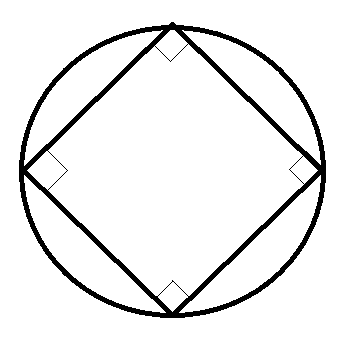If the area of the circle touching the square in the picture above is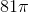, what is the closest value to the area of the square?

Possible Answers: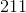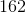Correct answer:Explanation:

Obtain the radius of the circle from the area.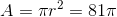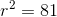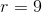Split the square up into 4 triangles by connecting opposite corners. These triangles will have a right angle at the center of the square, formed by two radii of the circle, and two 45-degree angles at the square's corners. Because you have a 45-45-90 triangle, you can calculate the sides of the triangles to be,, and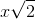. The radii of the circle (from the center to the corners of the square) will be 9. The hypotenuse (side of the square) must be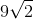.

The area of the square is then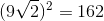.

### Example Question #1 : How To Find The Length Of The Diameter

Two legs of a right triangle measure 3 and 4, respectively. What is the area of the circle that circumscribes the triangle?

Possible Answers: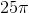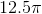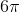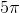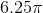Correct answer:Explanation:

For the circle to contain all 3 vertices, the hypotenuse must be the diameter of the circle. The hypotenuse, and therefore the diameter, is 5, since this must be a 3-4-5 right triangle.

The equation for the area of a circle is A = πr2.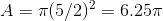### All High School Math Resources